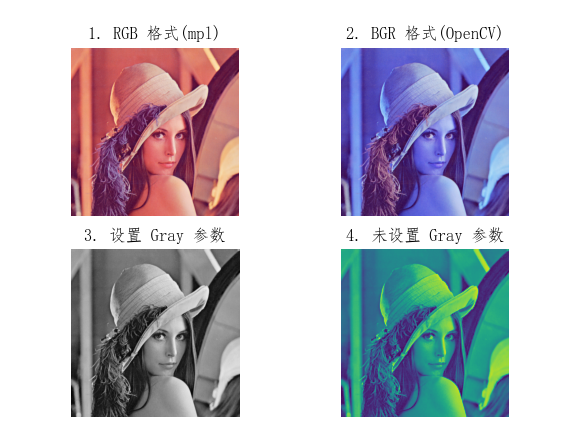# 【youcans 的 OpenCV 例程200篇】04. 用 matplotlib 显示图像（plt.imshow）

matplotlib.pyplot.imshow(img[, cmap])

OpenCV 使用 BGR 格式，matplotlib/PyQt 使用 RGB 格式。使用 matplotlib/PyQt 显示 openCV 图像，要将 BGR 格式转换为 RGB 格式：

``````# 图片格式转换：BGR(OpenCV) -> RGB(PyQt5)
imgRGB = cv2.cvtColor(imgBGR, cv2.COLOR_BGR2RGB)
``````

• img：图像数据，nparray 多维数组，对于 openCV（BGR）格式图像要先进行格式转换
• cmap：颜色图谱（colormap），默认为 RGB(A) 颜色空间
• gray：灰度显示
• hsv：hsv 颜色空间
• 注意事项：

1. OpenCV 和 matplotlib 中的彩色图像都是 Numpy 多维数组。但 OpenCV 使用 BGR 格式，颜色分量按照蓝/绿/红的次序排列，而 matplotlib 使用 RGB 格式，颜色分量按照红/绿/蓝的次序排序。因此用 plt.imshow() 显示 OpenCV 彩色图像时，先要进行颜色空间转换，将Numpy 多维数组按照红/绿/蓝的次序排序。
2. plt.imshow() 可以直接显示 OpenCV 灰度图像，不需要格式转换，但需要使用 cmap=‘gray’ 进行参数设置。
3. plt.imshow() 可以使用 matplotlib 库中的各种方法绘图，如标题、坐标轴、插值等，详见 matploblib Document
4. PyQt5 也使用 RGB 格式，因此在 PyQt5 中显示 OpenCV 彩色图像时，也要进行颜色空间转换。

基本例程：

``````    # 1.10 图像显示(plt.imshow)
imgFile = "../images/imgLena.tif"  # 读取文件的路径
img1 = cv2.imread(imgFile, flags=1)  # flags=1 读取彩色图像(BGR)

imgRGB = cv2.cvtColor(img1, cv2.COLOR_BGR2RGB)  # 图片格式转换：BGR(OpenCV) -> RGB(PyQt5)
img2 = cv2.cvtColor(img1, cv2.COLOR_BGR2GRAY)  # 图片格式转换：BGR(OpenCV) -> Gray

plt.rcParams['font.sans-serif'] = ['FangSong']  # 支持中文标签
plt.subplot(221), plt.title("1. RGB 格式(mpl)"), plt.axis('off')
plt.imshow(imgRGB)  # matplotlib 显示彩色图像(RGB格式)
plt.subplot(222), plt.title("2. BGR 格式(OpenCV)"), plt.axis('off')
plt.imshow(img1)    # matplotlib 显示彩色图像(BGR格式)
plt.subplot(223), plt.title("3. 设置 Gray 参数"), plt.axis('off')
plt.imshow(img2, cmap='gray')  # matplotlib 显示灰度图像，设置 Gray 参数
plt.subplot(224), plt.title("4. 未设置 Gray 参数"), plt.axis('off')
plt.imshow(img2)  # matplotlib 显示灰度图像，未设置 Gray 参数
plt.show()
``````

程序说明：

图 1 中 OpenCV 的 BGR 彩色图像已转换为 RGB 格式，彩色图像的颜色显示正常；
图 2 中 OpenCV 的 BGR 彩色图像格式未做转换，彩色图像的颜色显示异常；
图 3 中 plt.imshow() 设置 cmap=‘gray’，灰度图像的颜色显示正常；
图 4 中 plt.imshow() 未设置 cmap=‘gray’，灰度图像的颜色显示异常。（本节完）

版权声明：
youcans@xupt 原创作品，转载必须标注原文链接：(https://blog.csdn.net/youcans/article/details/125112487)